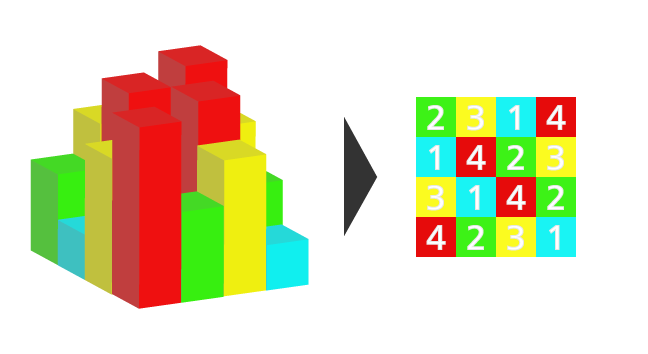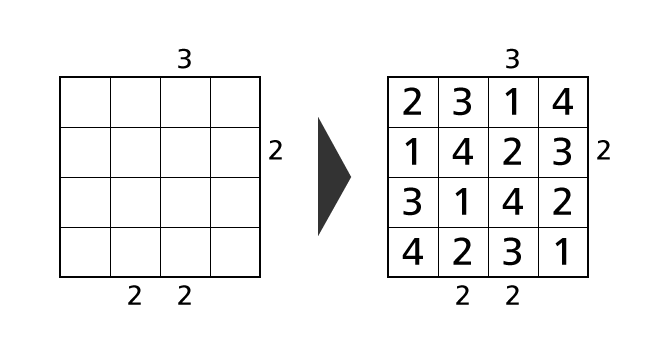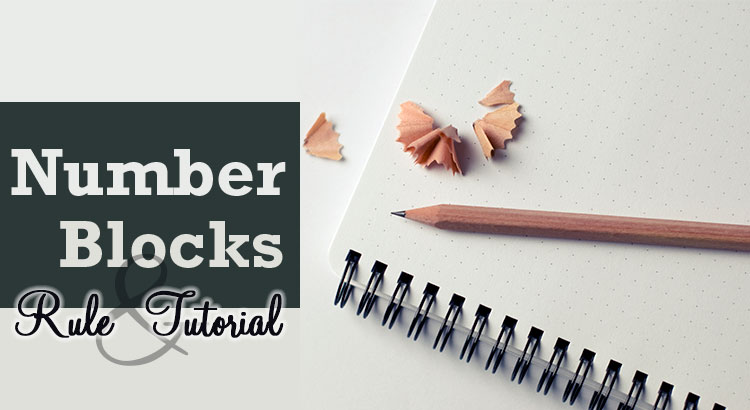## Introduction

When we play Skyscrapers Puzzle, we assume that we are watching the buildings with heights of 1–4 from above.Skyscrapers Puzzle is a puzzle game which we have to determine the place of numbers by using given hints under some rules.Here we explain the rule of Skyscrapers Puzzle with size 4×4 in detail for those who plays it for the first time. Then we demonstrate the solving process of an example puzzle.## Introduction

CalcBlocks is a puzzle game which we have to determine the place of numbers by using given hints under some rules. Here we explain the rule of CalcBlocks with size 4×4 in detail for those who plays it for the first time. Then we demonstrate the solving process of an example puzzle.

In explanations, we call a horizontal sequence of boxes row and a vertical sequence of boxes column. We describe a position of a box as (1,1) using a row and a column number. For example, we call the colored box in the following image box–(2,3).## Introduction

Sudoku 4×4 is a puzzle game which we have to determine the place of numbers by using given hints under some rules. Here we explain the rule of Sudoku 4×4 in detail for those who plays it for the first time. Then we demonstrate the solving process of an example puzzle.

In explanations, we call a horizontal sequence of boxes row and a vertical sequence of boxes column. We describe a position of a box as (1,1) using a row and a column number. For example, we call the colored box in the following image box–(2,3).